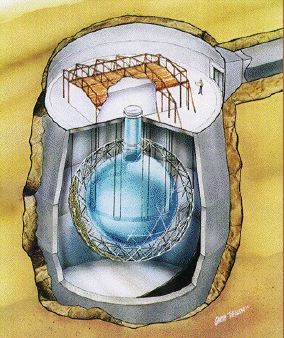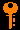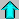# Lecture 15 - Stellar Energy (3/4/99)Star Formation --- | --- Stellar EvolutionReading:
Chapter 16-1, 16-2 (ZG4)

Notes:
pages 57 - 61

Artists conception of the Sudbury Neutrino Observatory. Observation of solar neutrinos can probe conditions at the very core of the Sun. (Courtesy SNO)
Key Question:## Investigations:

1. Stellar Energy
• What is the energy generation (Watts per kg) required to supply the luminosity of the Sun?
• What is the gravitational potential energy available in the Sun?
• How long could this supply the current solar luminosity?
• How much energy per nucleon is needed over the solar lifetime of 10 billion years?
• What is the binding energy of the electron in hydrogen? Could this supply the energy?
2. Nuclear Binding Energy
• What does the relativistic energy equation E = gamma*mc^2 imply?
• What is the rest mass of the proton (in MeV/c^2)? The electron?
• What is the approximate Coulomb barrier in a nucleus?
• Are there energy levels in the nucleus as in the electron orbitals?
• Can we extract energy from changes between these levels?
• What is the binding energy curve (E/nucleon vs. #nucleons) for atomic nuclei?
• When does fusion liberate energy? When does fission?
• How does the change in biniding energy show up in the mass?
• What is the mass defect of a helium-4 nucleus versus four protons?
• How much energy per nucleon can be extracted by fusion four protons to make a helium-4 nucleus?
• What fraction of the rest mass of a proton is converted into energy in this process?
• What fraction of the Sun's hydrogen must be fused into helium over its lifetime to supply the current luminosity?
3. The Proton-Proton Chain
• Approximately what is the thermal energy required to overcome the Coulomb barrier? What temperature does this correspond to?
• What is the main proton-proton chain (PP I)?
• What step takes the longest to occur? Why? What is the implication of this?
• What is the significance of neutrinos in the P-P reaction? How many are generated each second?
• What are the PP II and PP III chains?
• Where do high energy solar neutrinos come from?
• What is the solar neutrino problem?
• How do you detect solar neutrinos?
4. Other Fusion Chains
• At what temperature does the P-P reaction "turn on"?
• What is the CNO cycle? Why is it equivalent to P-P?
• At what temperature does CNO turn on? When does it dominate PP?
• What are the temperature dependences of PP and CNO?
• What is the triple-alpha process or helium burning?
• What are the temperature thresholds and reactions in carbon, oxygen, and silicon burning?
• How do you get heavier nuclei to fuse in a star?
• What happens when you try to fuse iron-peak elements (Fe, Co, Ni)?

## Solar Neutrinos:

Neutrino Astrophysics has a long tradition at Penn. There is a good discussion of the implications of the results of solar neutrino experiments set up by the group.

Some links to neutrino experiment sites:

## Stellar Energy in Outline:

1. Nuclear Energy in Stars
• We showed in previous lectures that chemical energy was insufficient to power the Sun. Even gravitational energy, which could provide the Sun's luminosity by shrinking by 40 meters every year, could only do so for millions of years, not the billions that the Sun has lasted.
• Since the gravitational and the electromagnetic forces are insufficient to provide the energy of the Sun, we turn to the remaining forces: the strong and weak nuclear forces. In particular, it is the strong nuclear force that can provide us this energy.
• The strong nuclear force is the force that holds protons and neutrons together in the nucleus of the atom, overcoming the mutual electrical repulsion of the protons. The strong force is carried by particles called gluons, which hold together the quarks that make up protons and neutrons. (Electrons are not made up of quarks - they are a different sort of particle called a lepton.)
• The weak nuclear force is a force that changes the kind of quark or lepton. The weak force is the kind of force that can change a proton into a neutron, and visa versa, for example.
• Just like we can emit a photon (energy) by moving an electron into a more tightly bound orbit, we can liberate nuclear energy in the form of photons and other particles by making the nucleus more tightly bound.
• It turns out that for elements ranging in mass from hydrogen to iron, the nuclei are more tightly bound as you increase the mass. For elements heavier than iron, the nuclei are less tightly bound the more massive they are.
• This means the for elements lighter than iron, you can release energy by the fusion of more than one together to make a heavier nucleus.
• Similarly, for elements heavier than iron, you can release energy by causing fission of a heavier nucleus into more than one lighter fragments.
• The way to measure the energy released in the fusion or fission of nuclei is to measure the mass of the nuclei before and after the reaction.
• Einstein's equation relates the mass and the equivalent energy:

E = m c^2

• If you could convert all of a proton's mass (1.673 x 10^-27 kg) into energy, you would get (1.67 x 10^-27 kg)(3 x 10^8 m/s)^2 = 1.506 x 10^-10 Joules. In more familiar units, this is equal to 938 x 10^6 eV or 938 MeV(millon-electron-volts). There is a tremedous amount of energy available in the mass of particles.
• For comparison, the mass of the electron gives 511 x 10^3 eV of energy, or 511 keV (kilo-electron-volts).
• Often the masses of particles are given in energy units of mc^2, so the proton mass is 938 MeV, and the electron mass is 511 keV. Note that you can also reverse the process, and given 938 MeV of energy you can create a proton! (It turns out you can't just create a proton, you have to create an anti-proton also. We will discuss this later on).
• Thus, if you take four hydrogen nuclei (protons) and convert them into one helium nucleus (2 proton, 2 neutrons), you find that the sum of the 4 proton masses is 0.7% larger than the mass of 1 helium nucleus.
• This difference in mass comes out in energy (photons and particles). The amount of energy is calculated by E = m c^2, where m is the mass difference, in this case 4.8 x 10^-29 kg which gives 27 MeV of energy. Compare this with the 50 eV or so of electromagnetic energy available from the ionization energy of helium.
• It is hard to get the repulsive protons close enough together to let the strong force take over. You need very high temperatures (T > 10^7 K) in order for strong collisions to cause these reactions to happen. This is why this occurs in the very cores of stars that are massive enough to be that hot!
• The reaction that supplies the energy for the Sun is:

4 H -> He

• The main way of doing this is through the proton-proton reaction. This occurs in 3 stages:

H + H -> D + e+ + v (x2 , 1.4 x 10^10 yrs)
H + D -> He3 + photon (x2 , 6 seconds)

H + He3 -> He + H + H (x1 , 10^6 yrs)

The funny things in the first reaction are a positron (e+), which is the anti-electron and is just like an electron but positively charged, and the neutrino (v), which is a very light particle. Note that the first reaction is very slow to happen, taking over 10 billion years on average. This is because it turns a proton into a neutron (D is deuterium a kind of hydrogen consisting of a proton and a neutron bound together) using the weak force (hence the positron and neutrino). The other reactions only use the strong force, and are easier.
• This reaction provides 90% of the Sun's energy. It requires temperatures above 10 million K (10^7 K).
2. Other Nuclear Reactions
• The CNO cycle (for Carbon - Nitrogen - Oxygen) also turns 4 H -> He, but using carbon as a catalyst. This provides about 10% of the energy of the Sun, and requires temperatures of 16 million K.
• The Triple Alpha reaction turns 3 He -> C ("alpha particles" are another term for the helium nucleus). This reaction needs temperatures above 100 million K, and do not happen in the Sun at this time. Later, however ...
• Carbon Fusion can occur above about 600 million K, and produces oxygen, neon, magnesium and silicon. At still higher temperatures around 3.5 billion K, silicon can be fused to form heavier elements, including iron.
• The iron barrier: if you remember our consideration of the binding energy per nucleon of the nucleus as a function of the atomic weight of the nucleus, we showed that you could extract energy from fusion only for elements lighter than iron. Thus, iron is the "end of the line" for fusion as an energy generator.Prev Lecture ---Next Lecture ---Astr12 Index ---Astr12 Home

smyers@nrao.edu Steven T. Myers